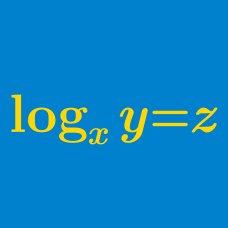Algebra

# Logarithmic Functions - Solving Equations

If $x$ satisfies the equation $\log{x} = \log{322} - \frac{\log{49}}{2}$, what is the value of $x$?

Suppose that $0 \leq x \leq \pi$ satisfies $\log_{\sin x}{\cos x} + \log_{\cos x}{\tan x} = 1.$ If $x = \frac{a}{b}\pi$, where $a$ and $b$ are coprime positive integers, what is the value of $a+b$?

The two roots of the quadratic equation for $x$ $x^2-(ab-14)x + a^{\log_2 b} - 32 = 0$ are $16$ and $2$, where $0. What is the value of $a + b$?

$x$ and $y$ are real numbers that satisfy the following two equations: $3^x \cdot 2^y = 3456 \;\;\; \textrm{and} \;\;\; \log_{\sqrt{2}} (y-x) = 4.$ What is the value of $x + y$?

What is the sum of all real values of $x$ that satisfy $3 \log_x 2 + \log_{2} x = 4 ?$

×